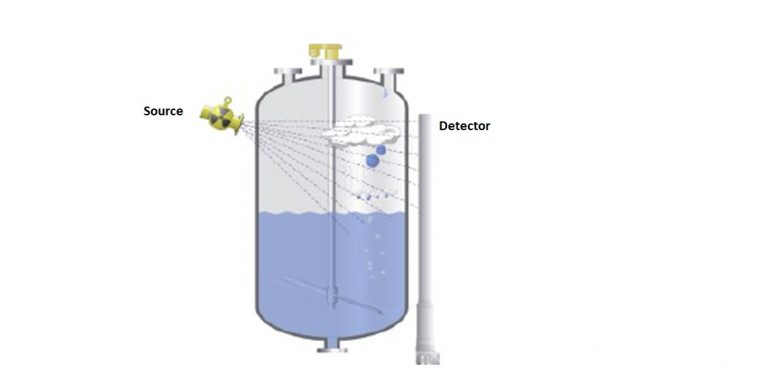# Density Measurement

## What is Density?

The densityρ, of an object is defined as the ratio of its mass to its volume. Density can be useful in identifying substances. It is also a convenient property because it provides a link (or conversion factor) between the mass and the volume of a substance.

ρ = m/V

Mass and volume are extensive (or extrinsic) properties of matter – they depend on amount. Density, an intensive (or intrinsic) property, is a kind of “heaviness” factor. In macroscopic terms, density reflects how much mass is packed into a given three-dimensional space. Typically, densities are reported g/ml or g/cm3 (which are equivalent because 1ml ≡ 1cm3). Experimentally, mass and volume measurements are required to calculate density.

### Method of Density Measurement :-

Density can be measured by following method

• Hydrostatic pressure
• Vibration
• Differential pressure

#### Hydrostatic pressure Method:-

The density (ρ) of an object or material sample is equal to its mass (m) divided by its volume (V).

ρ = m/V

The specific gravity (SG) of a material is equal to its density divided by the density of pure water. The specific gravity is greater than one for materials that are denser than water and less than one for materials that are less dense than water.

Hydrostatic Fluid Pressure The following very general formula can be used to calculate the change in pressure (Δp) associated with a specific change in depth (Δh) in any incompressible fluid having density (ρfluid) subjected to any constant acceleration (a). For example, this formula can be used to calculate pressure changes under an ocean of liquid water on Europa (one of Jupiter’s moons) or even under the sea of liquid methane thought to exist on Titan (one of Saturn’s moons).

Δp = ρfluid aΔh

In the specific case of water on or near earth’s surface, where the freefall acceleration due to gravity is g = 9.8 m/s2 , this becomes:

Δp = ρH2O gΔh

For additional information about calculating hydrostatic pressure on earth, including tables of pressure as a function of depth in both freshwater and seawater.

The radiometric measurement of density is based on the Gamma transmission principle. Gamma radiation is attenuated as it passes through a pipeline. The extent to which the radiation is attenuated is dependent on the density of the medium in the pipeline. The higher the density, the less radiation reaches the detector.#### Vibration method:-

While many methods for measuring the fluid density are known, they are often time consuming. Density determination based on the hydrostatic principle is known to achieve the highest accuracy for measuring the density of liquids. Apart from long measurement time, it requires a large amount of the liquid to be measured. A vibrating tube density meter is based on a bent tube that is filled with the liquid to be measured. The tube is brought to resonant oscillation by exerting a suitable force on the tube. The liquid under test changes the mass of the system and hence the resonance frequency of the glass tube. Since the volume of the glass tube is kept constant, there is a direct relationship between the measured resonance frequency and the density of the liquid.

#### Differential pressure Method:

In density measurement of liquids with differential pressure, two pressure measuring points are mounted at a fixed distance from each other. There are thus two different pressures acting on the system. The differential pressure transducer calculates the density of the medium from the resulting pressure difference.

Related Search:-